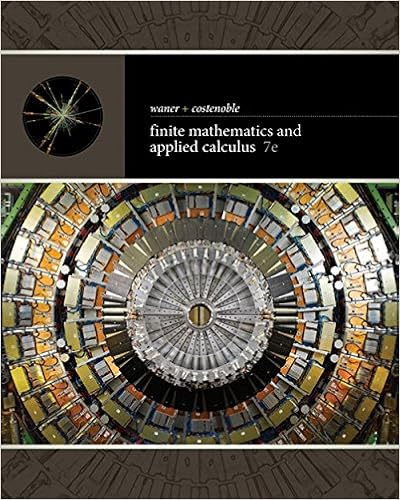# Demand rises by the same amount that supply falls

• 4
• 100% (2) 2 out of 2 people found this document helpful

This preview shows page 1 - 3 out of 4 pages.

##### We have textbook solutions for you!
The document you are viewing contains questions related to this textbook.The document you are viewing contains questions related to this textbook.
Chapter 12 / Exercise 7
Finite Mathematics and Applied Calculus
Costenoble/WanerExpert Verified
F.Demand falls by the same amount that supply rises = Equilibrium price falls and
##### We have textbook solutions for you!
The document you are viewing contains questions related to this textbook.The document you are viewing contains questions related to this textbook.
Chapter 12 / Exercise 7
Finite Mathematics and Applied Calculus
Costenoble/WanerExpert Verified
3. Draw a diagram that shows a larger increase in demand than the decrease in supply.
4.Draw a diagram that shows a smaller increase in supply than the increase in demand.See drawing attached.II. Chapter 4: Questions & Problems: 9 (p122)Working with Numbers and Graphs: 2 (p122)9. What is the difference between a price ceiling and a price floor? What effect is the same for both a price ceiling and a price floor?
•••